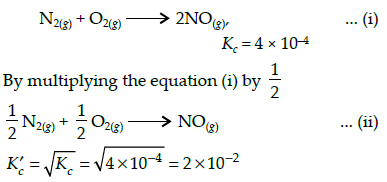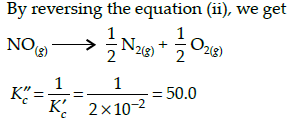Courses

# Test: Previous Year Questions - Chemical Equilibrium (Level 1)

## 17 Questions MCQ Test Chemistry Class 11 | Test: Previous Year Questions - Chemical Equilibrium (Level 1)

Description
This mock test of Test: Previous Year Questions - Chemical Equilibrium (Level 1) for Class 11 helps you for every Class 11 entrance exam. This contains 17 Multiple Choice Questions for Class 11 Test: Previous Year Questions - Chemical Equilibrium (Level 1) (mcq) to study with solutions a complete question bank. The solved questions answers in this Test: Previous Year Questions - Chemical Equilibrium (Level 1) quiz give you a good mix of easy questions and tough questions. Class 11 students definitely take this Test: Previous Year Questions - Chemical Equilibrium (Level 1) exercise for a better result in the exam. You can find other Test: Previous Year Questions - Chemical Equilibrium (Level 1) extra questions, long questions & short questions for Class 11 on EduRev as well by searching above.
QUESTION: 1

### For the reaction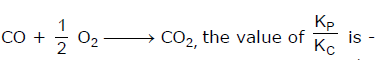[AIEEE-2002]

Solution:

The correct answer is option C
Kp = Kc (RT)Δn;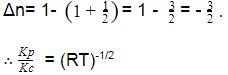QUESTION: 2

### Which of the following equilibria is not affected by change in volume of the flask [AIEEE-2002]

Solution:

Change in volume affects number of moles per unit volume and move in the direction which undo the change.

N2(g)+ O2(g) ⇄2NO(g)

Number of moles of reactants and products are equal.

QUESTION: 3

### For the reaction equilibrium N2O4 (g) ⇌ 2NO2 (g) the concentrations of N2O4 and NO2 at equilibrium are 4.8 × 10-2 and 1.2 × 10-2 mol L-1 respectively. The value of KC for the reaction is -        [AIEEE-2003]

Solution: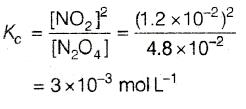QUESTION: 4

Consider the reaction equilibrium  2SO2(g)+O2(g)2 SO3 (g); ΔHº = -198 kJ

On the basis of Le Chatelier's principle, the condition favourable for the forward reaction is -

[AIEEE-2003]

Solution:

The correct answer is option A

ACCORDING TO LE CHATELIER'S PRINCIPLE

TEMPERATURE
if we increase temperature equilibrium will shift that direction which proceeds an endothermic reaction but the reaction given in question is exothermic so we have to decrease temperature to proceed forward.

PRESSURE
at equilibrium if we increase pressure equilibrium will shift that direction which has less number of moles but in given reaction products have less no. of moles than reactant that's why we increase pressure.

QUESTION: 5

What is the equilibrium expression for the reaction P4(S) + 5O2(g)  P4O10(s) ?

[AIEEE-2004]

Solution:

In the expression for equilibrium constant (Kp or Kc) species  state are not written (i.e., their molar concentrations are not taken as 1)
P4 (s) + 5O2 (g) ⇌ P4O10 (s)
Thus, Kc = 1/[O2]5

QUESTION: 6

For the reaction CO(g) + Cl2(g) COCl2(g) the KP/KC is equal to -

[AIEEE-2004]

Solution:

Kp =Kc (RT)Δn
Δn = sum of coefficients of gaseous products
Sum of coefficients of gaseous reactants.
CO (g) + Cl2 (g) → COCl2 (g)
Δn = 1-2 = -1
Kp = Kc (RT)-1
Kp/Kc = (RT)-1
1/RT

QUESTION: 7

The equilibrium constant for the reaction N2(g) + O2(g) 2NO(g) at temperature T is 4×10-4. The value of KC for the reaction NO(g)  1/2 N2(g) + O2(g) at the same temperature is-

[AIEEE-2004]

Solution: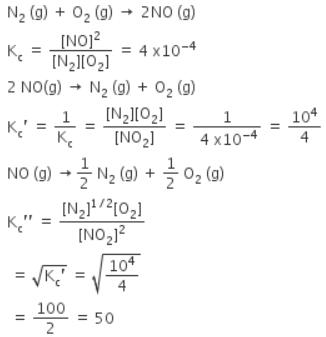QUESTION: 8

For the reaction 2 NO2(g) 2 NO(g) + O2(g),

(Kc = 1.8 × 10_6 at 184ºC)

(R = 0.0831 kJ/(mol.K))

When Kp and Kc are compared at 184ºC it is found that

[AIEEE-2005]

Solution: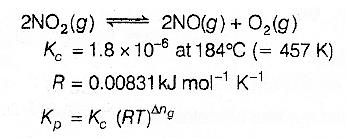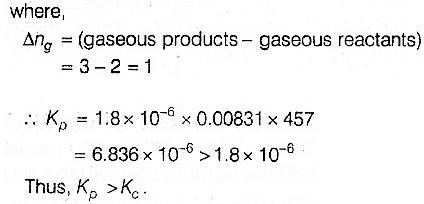QUESTION: 9

The exothermic formaton of ClF3 is represented by the equation -

Cl2(g)+3F2(g) 2ClF3(g) ; ΔrH = -329 kJ

Which of the following will increase the quantity of ClF3 in an equilibrium mixture of Cl2, F2 and ClF3 ?

Solution:

The correct answer is option C
According to Le-Chatelier's Principle, if a system at equilibrium is subjected to a change of concentration pressure or temperature then the equilibrium is shifted in such a way as to nullify the effect of change.
In the given reaction, if the concentration of F2 is increased then the reaction will shift in the forward direction in order to increase the concentration of ClF3.
Hence,

QUESTION: 10

A schematic plot of ln Keq versus inverse of temperature for a reaction is shown below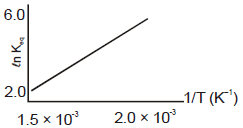The reaction must be

Solution: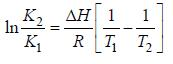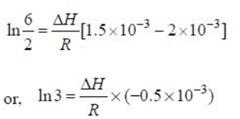ΔH Of reaction comes out to be negative. Hence reaction is exothermic.

QUESTION: 11

An amount of solid NH4HS is placed in a flask already containing ammonia gas at a certain temperature and 0.50 atm pressure. Ammonium hydrogen sulphide decomposes to yield NH3 and H2S gases in the flask. When the decomposition reaction reaches equilibrium, the total pressure in the flask rises to 0.84 atm ? The equilibrium constant for NH4HS decomposition at this temperature is

[AIEEE-2005]

Solution: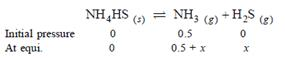Total pressure = 0.5 + 2x = 0.84 .. x = 0.17 atm.
Kp =PNH3 x PH2s = (0.5 + 0.17)(0.17)=0.11 atm2.

QUESTION: 12

Phosphorus pentachloride dissociates as follows, is a closed reaction vessel,

PCl5(g) PCl3(g) + Cl2(g)

If total pressure at equilibrium of the reaction mixture is P and degree of dissociation of PCl5 is x, the partial pressure of PCl3 will be

[AIEEE 2006]

Solution: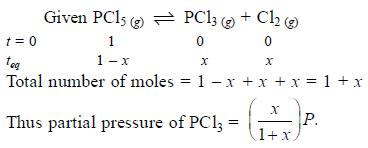QUESTION: 13

The equilibrium constant for the reaction

SO3(g) SO2(g) + 1/2 O2(g) is Kc = 4.9×10-2. The value of Kc for the reaction

2SO2(g)+O2(g) 2SO3(g) will be

[AIEEE 2006]

Solution: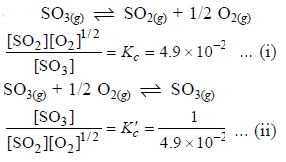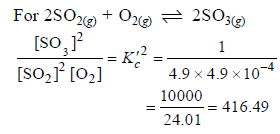QUESTION: 14

For the following three reactions a, b and c, equilibrium constants are given

(a) CO(g) + H2O(g) CO2(g) +H2 (g); K1

(b) CH4(g) + H2O(g) CO(g) + 3H2(g); K2

(c) CH4(g) + 2H2O(g) CO2(g) + 4H2(g); K3

Which of the following relations is correct

Solution:

Equation (c) = equation (a) + equation (b) Thus K3 = K1.K2

QUESTION: 15

The equilibrium constants Kp1 and Kp2 for the reactions X  2Y and Z P + Q, respectively are in the ratio of 1 : 9. If the degree of dissociation of X and Z be equal then the ratio of total pressures at these equilibria is -

[AIEEE 2008]

Solution: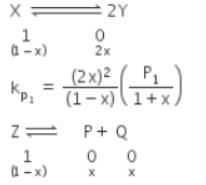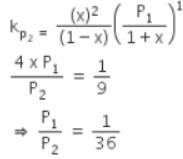QUESTION: 16

A vessel at 1000 K contains CO2 with a pressure of 0.5 atm. Some of the CO2 is converted into CO on the addition of graphite. If the total pressure at equilibrium is 0.8 atm, the value of Kp is

[AIEEE 2011]

Solution:

Kp depends upon the partial pressure of reactants and products so first calculate their partial pressure and then, calculate Kp,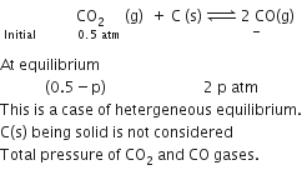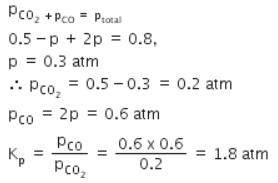QUESTION: 17

The equilibrium constant (Kc) for the reaction N2(g)+O2(g) → 2NO(g) at temperature T is

4×10-4. The value of Kc for the reaction,
NO(g)→ ½ N2(g) + ½O2(g) at the same temperature is:

[AIEEE 2012]

Solution: